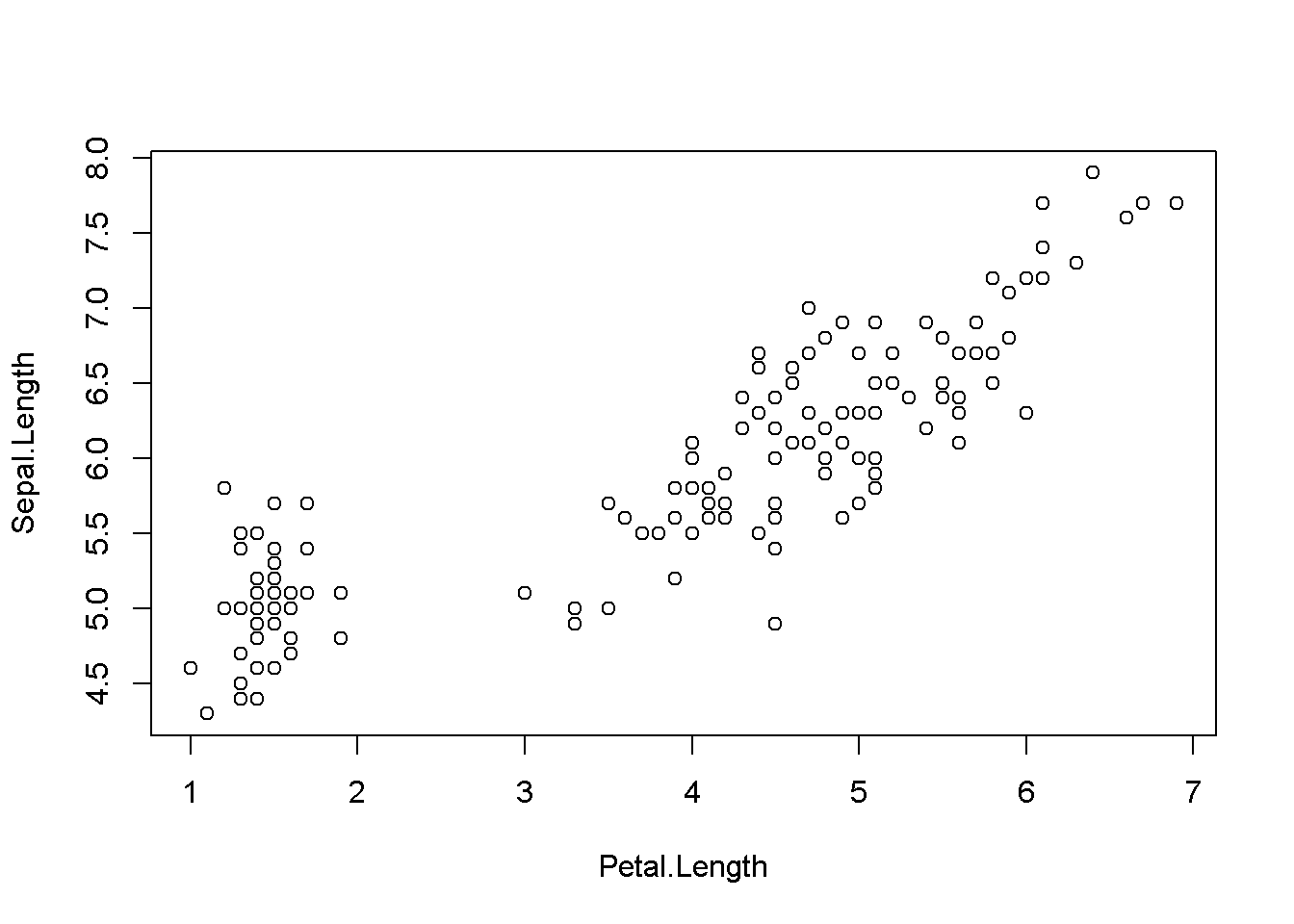Some notes on adjusting margins and using the mtext() function to place axis labels closer the axes.

Standard plot 1 panel plot

Issues

• Large margin above the plot
• Large distance between labels on the tick marks and the axis label
data(iris)
with(iris, plot(Sepal.Length ~ Petal.Length))Change margins around plot

• use “par(mar = c(lower, left,top,right))”
• defult is mar = c(5, 4, 4, 2) + 0.1.

Here, I decrease the top and left margins to 1.

par(mar = c(5,4,1,1))
with(iris, plot(Sepal.Length ~ Petal.Length))